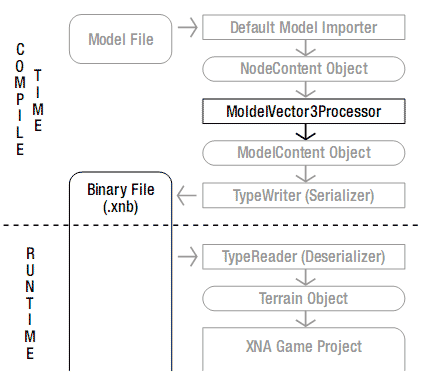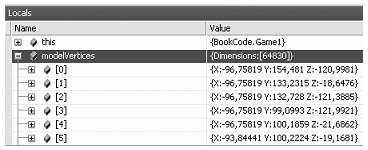# 4.13 通过扩展模型处理器直接访问顶点位置数据

## 解决方案## 工作原理

[ContentProcessor]
public class ModelVector3Processor : ModelProcessor
{
public override ModelContent Process(NodeContent input, ContentProcessorContext context)
{
ModelContent usualModel = base.Process(input, context);
List<Vector3> vertices = new List<Vector3>();
usualModel.Tag = vertices.ToArray();
return usualModel;
}
} 

private List<Vector3> AddVerticesToList(NodeContent node, List<Vector3> vertList)
{
MeshContent mesh = node as MeshContent;
if (mesh != null)
{
//This node contains vertices, add them to the list
}
foreach (NodeContent child in node.Children)
return vertList;
} 

Matrix absTransform = mesh.AbsoluteTransform;
foreach (GeometryContent geo in mesh.Geometry)
{
foreach (int index in geo.Indices)
{
Vector3 vertex = geo.Vertices.Positions[index];
Vector3 transVertex = Vector3.Transform(vertex, absTransform);
}
} 

myModel = content.Load<Model>("tank");
modelTransforms = new Matrix[myModel.Bones.Count];
Vector3[] modelVertices = (Vector3[])myModel.Tag;
System.Diagnostics.Debugger.Break();## 代码

namespace Vector3Pipeline
{
[ContentProcessor]
public class ModelVector3Processor : ModelProcessor
{
public override ModelContent Process(NodeContent input, ContentProcessorContext context)
{
ModelContent usualModel = base.Process(input, context);
List<Vector3> vertices = new List<Vector3>();
usualModel.Tag = vertices.ToArray();
return usualModel;
}

private List<Vector3> AddVerticesToList(NodeContent node, List<Vector3> vertList)
{
MeshContent mesh = node as MeshContent;
if (mesh != null)
{
Matrix absTransform = mesh.AbsoluteTransform;
foreach (GeometryContent geo in mesh.Geometry)
{
foreach (int index in geo.Indices)
{
Vector3 vertex = geo.Vertices.Positions[index];
Vector3 transVertex = Vector3.Transform(vertex, absTransform);
}
}
}

foreach (NodeContent child in node.Children)
}